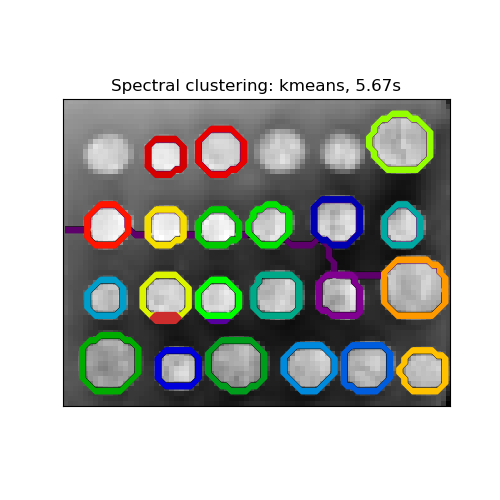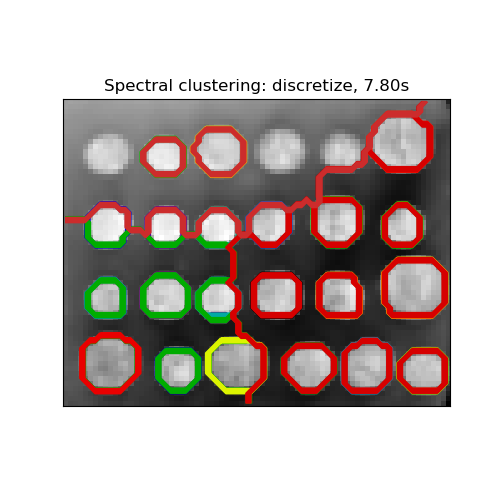# Segmenting the picture of greek coins in regions¶

This example uses Spectral clustering on a graph created from voxel-to-voxel difference on an image to break this image into multiple partly-homogeneous regions.

This procedure (spectral clustering on an image) is an efficient approximate solution for finding normalized graph cuts.

There are two options to assign labels:

• with ‘kmeans’ spectral clustering will cluster samples in the embedding space using a kmeans algorithm
• whereas ‘discrete’ will iteratively search for the closest partition space to the embedding space.
print(__doc__)

# Author: Gael Varoquaux <gael.varoquaux@normalesup.org>, Brian Cheung
# License: BSD 3 clause

import time

import numpy as np
from scipy.ndimage.filters import gaussian_filter
import matplotlib.pyplot as plt
from skimage.data import coins
from skimage.transform import rescale

from sklearn.feature_extraction import image
from sklearn.cluster import spectral_clustering

# load the coins as a numpy array
orig_coins = coins()

# Resize it to 20% of the original size to speed up the processing
# Applying a Gaussian filter for smoothing prior to down-scaling
# reduces aliasing artifacts.
smoothened_coins = gaussian_filter(orig_coins, sigma=2)
rescaled_coins = rescale(smoothened_coins, 0.2, mode="reflect")

# Convert the image into a graph with the value of the gradient on the
# edges.
graph = image.img_to_graph(rescaled_coins)

# Take a decreasing function of the gradient: an exponential
# The smaller beta is, the more independent the segmentation is of the
# actual image. For beta=1, the segmentation is close to a voronoi
beta = 10
eps = 1e-6
graph.data = np.exp(-beta * graph.data / graph.data.std()) + eps

# Apply spectral clustering (this step goes much faster if you have pyamg
# installed)
N_REGIONS = 25


Out:

/home/circleci/miniconda/envs/testenv/lib/python3.6/site-packages/skimage/transform/_warps.py:23: UserWarning: The default multichannel argument (None) is deprecated.  Please specify either True or False explicitly.  multichannel will default to False starting with release 0.16.
warn('The default multichannel argument (None) is deprecated.  Please '


Visualize the resulting regions

for assign_labels in ('kmeans', 'discretize'):
t0 = time.time()
labels = spectral_clustering(graph, n_clusters=N_REGIONS,
assign_labels=assign_labels, random_state=42)
t1 = time.time()
labels = labels.reshape(rescaled_coins.shape)

plt.figure(figsize=(5, 5))
plt.imshow(rescaled_coins, cmap=plt.cm.gray)
for l in range(N_REGIONS):
plt.contour(labels == l,
colors=[plt.cm.nipy_spectral(l / float(N_REGIONS))])
plt.xticks(())
plt.yticks(())
title = 'Spectral clustering: %s, %.2fs' % (assign_labels, (t1 - t0))
print(title)
plt.title(title)
plt.show()

••Out:

Spectral clustering: kmeans, 5.67s
Spectral clustering: discretize, 7.80s


Total running time of the script: ( 0 minutes 14.510 seconds)

Gallery generated by Sphinx-Gallery